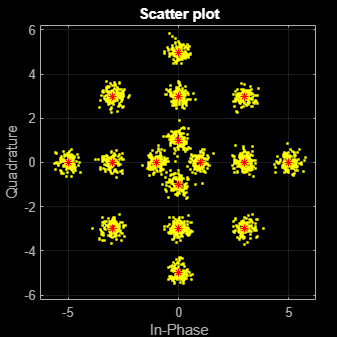# awgn

Add white Gaussian noise to signal

## Syntax

``out = awgn(in,snr)``
``out = awgn(in,snr,signalpower)``
``out = awgn(in,snr,signalpower,randobject) ``
``out = awgn(in,snr,signalpower,seed)``
``out = awgn(___,powertype)``

## Description

````out = awgn(in,snr)` adds white Gaussian noise to the vector signal `in`. This syntax assumes that the power of `in` is 0 dBW.```

example

````out = awgn(in,snr,signalpower)` accepts an input signal power value in dBW. To have the function measure the power of `in` before adding noise, specify `signalpower` as `'measured'`.```

example

````out = awgn(in,snr,signalpower,randobject) ` accepts input combinations from prior syntaxes and a random number stream object to generate normal random noise samples. For information about producing repeatable noise samples, see Tips.```
````out = awgn(in,snr,signalpower,seed)` specifies a seed value for initializing the normal random number generator that is used when adding white Gaussian noise to the input signal. For information about producing repeatable noise samples, see Tips.```
````out = awgn(___,powertype)` specifies the signal and noise power type as `'dB'` or `'linear'` in addition to the input arguments in any of the previous syntaxes.For the relationships between SNR and other measures of the relative power of the noise, such as Es/N0, and Eb/N0, see AWGN Channel Noise Level.```

## Examples

collapse all

Create a sawtooth wave.

```t = (0:0.1:10)'; x = sawtooth(t);```

Apply white Gaussian noise and plot the results.

```y = awgn(x,10,'measured'); plot(t,[x y]) legend('Original Signal','Signal with AWGN')```Transmit and receive data using a nonrectangular 16-ary constellation in the presence of Gaussian noise. Show the scatter plot of the noisy constellation and estimate the symbol error rate (SER) for two different signal-to-noise ratios.

Create a 16-QAM constellation based on the V.29 standard for telephone-line modems.

```c = [-5 -5i 5 5i -3 -3-3i -3i 3-3i 3 3+3i 3i -3+3i -1 -1i 1 1i]; M = length(c);```

Generate random symbols.

`data = randi([0 M-1],2000,1);`

Modulate the data by using the `genqammod` function. General QAM modulation is necessary because the custom constellation is not rectangular.

`modData = genqammod(data,c);`

Pass the signal through an AWGN channel having a 20 dB signal-to-noise ratio (SNR).

`rxSig = awgn(modData,20,'measured');`

Display a scatter plot of the received signal and the reference constellation, `c`.

```h = scatterplot(rxSig); hold on scatterplot(c,[],[],'r*',h) grid hold off```Demodulate the received signal by using the `genqamdemod` function. Determine the number of symbol errors and the symbol error ratio.

```demodData = genqamdemod(rxSig,c); [numErrors,ser] = symerr(data,demodData)```
```numErrors = 1 ```
```ser = 5.0000e-04 ```

Repeat the transmission and demodulation process with an AWGN channel having a 10 dB SNR. Determine the symbol error rate for the reduced SNR. As expected, the performance degrades when the SNR is decreased.

```rxSig = awgn(modData,10,'measured'); demodData = genqamdemod(rxSig,c); [numErrors,ser] = symerr(data,demodData)```
```numErrors = 462 ```
```ser = 0.2310 ```

Generate white Gaussian noise addition results using a `RandStream` object and the `reset` object function.

Specify the power of X to be `0` dBW, add noise to produce an SNR of `10 `dB, and utilize a local random stream.

```S = RandStream('mt19937ar','Seed',5489); sigin = sqrt(2)*sin(0:pi/8:6*pi); sigout1 = awgn(sigin,10,0,S);```

Add AWGN to `sigin`. Use `isequal` to compare `sigout1` to `sigout2`. The outputs are not equal when the random stream was not reset.

```sigout2 = awgn(sigin,10,0,S); isequal(sigout1,sigout2)```
```ans = logical 0 ```

Reset the random stream object, returning the object to its state prior to adding AWGN to `sigout1`. Add AWGN to `sigin` and compare `sigout1` to `sigout3`. The outputs are equal after the random stream was reset.

```reset(S); sigout3 = awgn(sigin,10,0,S); isequal(sigout1,sigout3)```
```ans = logical 1 ```

## Input Arguments

collapse all

Input signal, specified as a scalar, vector, or array. The power of the input signal is assumed to be 0 dBW.

Data Types: `double`
Complex Number Support: Yes

Signal-to-noise ratio in dB, specified as a scalar.

Note

When the noise is added, this function applies the same `snr` to all elements of the full input signal. Array input signals do not have a notion of independent channels. To consider multiple channels independently, see `comm.AWGNChannel`.

Data Types: `double`

Signal power, specified as a scalar or `'measured'`.

• When `signalpower` is a scalar, the value is used as the signal level of `in` to determine the appropriate noise level based on the value of `snr`.

• When `signalpower` is `'measured'`, the signal level of `in` is computed to determine the appropriate noise level based on the value of `snr`.

Note

When you specify `'measured'`, this function computes the signal power using all elements of the full input signal. When the power is computed, array input signals do not have a notion of independent channels.

Data Types: `double`

Random number stream object, specified as a `RandStream` object. The state of the random stream object determines the sequence of numbers produced by the `randn` function. Configure the random stream object using the `reset (RandStream)` function and its properties.

`wgn` generates normal random noise samples using `randn`. The `randn` function uses one or more uniform values from the `RandStream` object to generate each normal value.

For information about producing repeatable noise samples, see Tips.

Random number generator seed value, specified as a scalar.

Data Types: `double`

Signal power unit, specified as `'dB'` or `'linear'`

• When `powertype` is `'dB'`, the `snr` is measured in dB and `signalpower` is measured in dBW.

• When `powertype` is `'linear'`, the `snr` is measured as a ratio and `signalpower` is measured in watts.

For the relationships between SNR and other measures of the relative power of the noise, such as Es/N0, and Eb/N0, see AWGN Channel Noise Level.

## Output Arguments

collapse all

Output signal, returned as a scalar, vector, or array. The returned output signal is the input signal with white Gaussian noise added to it.

## Extended Capabilities

### Topics

Introduced before R2006a